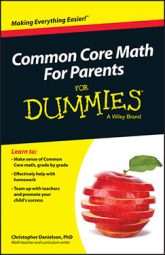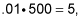##### Common Core Math For Parents For Dummies with Videos OnlineIn a Common Core classroom, seventh grade students use algebra to describe their understanding of mathematical relationships in the world. They also learn to state patterns and structure that appear in their arithmetic, and to notice and state new relationships.

In seventh grade, most of these relationships are proportional, which means that there is a constant rate of change in one variable relative to another.

Students' experiences with arithmetic and with real‐world mathematical relationships (such as interest) are the basis for learning algebra in a Common Core classroom. This is a far cry from the decoding of complicated and meaningless word puzzles that has cluttered algebra classrooms for many years.

Story problems or word problems — it doesn't matter what you call them — have been the heart of algebra teaching for many years and a source of anxiety for many students. Problems, such as the following one, force students to ask when they are ever going to use algebra.

Lucie bought 36 apples at the grocery store. She bought three times as many red apples as green apples, and twice as many green apples as yellow apples. How many yellow apples did she buy?

Seventh graders know that there is no possible scenario in which Lucie would know that she bought twice as many green apples as yellow apples without knowing how many of each she bought. Some students view these problems as fun little puzzles. But many students take these problems as a sign that algebra is not useful for much of anything.

A Common Core classroom has fewer of these silly word problems. Instead, algebra involves looking for the mathematical structure in everyday situations and in the arithmetic with which students are familiar. In short, students learn introductory algebra as an extension of what they already know.

For example, seventh graders already know how to find 1 percent of the money in a savings account (a realistic interest rate) in order to calculate the value of the account after a year. Using their knowledge from elementary grades, students are likely to multiply .01 by the balance of the account, and then add the result to the balance.

Doing this with a \$500 bank account goes like this:then 500 + 5 = 505. Seventh graders use algebra in two ways to describe what is happening.

• They notice that the procedure is the same no matter what the beginning balance is in the account. Nothing was special about 500, so students can use any number and the procedures are the same. Students can write the general relationship using variables. In this case, they can write x for each instance of the number 500, like this: x + .01x.

• When students use the distributive property to rewrite x + .01x as (1 + .01)x, or 1.01x, they're making an important algebraic leap. Writing x + .01x makes it difficult to calculate two years worth of interest. When you try to write the expression, things get complicated. But writing it as 1.01x, each extra year just requires one more set of parentheses. Two years of interest looks like this: 1.01(1.01x) because x is the initial balance and 1.01x is the balance after one year, which means 1.01x is the initial balance for the second year and so on. This particular relationship forms the foundation for exponential functions in high school, but the principle applies everywhere in the algebra curriculum.